Interpretable Aide Diagnosis System for Melanoma Recognition | OMICS International
Journal of Bioengineering & Biomedical Science

Like us on:

All submissions of the EM system will be redirected to Online Manuscript Submission System. Authors are requested to submit articles directly to Online Manuscript Submission System of respective journal.

# Interpretable Aide Diagnosis System for Melanoma Recognition

Messadi M1*, Ammar M2, Cherifi H1, Chikh MA2 and Bessaid A2

1Laboratoire Electronique, Informatique et Image UMR CNRS 6306, Université de Bourgogne, France

2Biomedical Engineering Laboratory, Department of Electrical and Electronics, Technology Faculty, Abou Bekr Belkaid, Tlemcen University – 13000, Algeria

*Corresponding Author:
Laboratoire Electronique
Informatique et Image UMR CNRS 6306
Université de Bourgogne, France
Tel: 043202336
E-mail: [email protected]

Received Date: August 10, 2014; Accepted Date: September 10, 2014; Published Date: September 20, 2014

Citation: Messadi M, Ammar M, Cherifi H, Chikh MA, Bessaid A (2014) Interpretable Aide Diagnosis System for Melanoma Recognition. J Bioengineer & Biomedical Sci 4: 132. doi: 10.4172/2155-9538.1000132

Copyright: © 2014 Messadi M, et al. This is an open-access article distributed under the terms of the Creative Commons Attribution License, which permits unrestricted use, distribution, and reproduction in any medium, provided the original author and source are credited.

Visit for more related articles at Journal of Bioengineering & Biomedical Science

#### Abstract

During the last years, computer vision-based diagnosis systems have been widely used in several hospitals and dermatology clinics, aiming mostly at the early detection of malignant melanoma tumor, which is among the most frequent types of skin cancer, versus other types of non-malignant cutaneous diseases. The mortality rate can be decreased by earlier detection of suspicious lesions and better prevention. The aim of this paper is to propose an interpretable classification method for skin tumors in dermoscopic images based on shape descriptors. This work presents a fuzzy rule based classifier to discriminate a melanoma. An adaptive Neuro Fuzzy inference System (ANFIS) is applied in order to discover the fuzzy rules leading to the correct classification. In the first step of the proposed work, we apply the Dullrazor technique to reduce the influence of small structures, hairs, bubbles, light reflexion. In the second step, an unsupervised approach for lesion segmentation is proposed. Iterative thresholding is applied to initialize level set automatically. In this paper, we have also treated the necessity to extract all the specific attributes used to develop a characterization methodology that enables specialists to take the best possible diagnosis. For this purpose, our proposal relies largely on visual observation of the tumor while dealing with some characteristics such as color, texture or form. The method used in this paper is called ABCD. It requires calculating 4 factors: Asymmetry (A), Border (B), Color (C) and Diversity (D). These parameters are used to construct a classification module based on ANFIS for the recognition of malignant melanoma. Finally, we compare the results of classification obtained by ANFIS with SVM (support vector machine) and artificial neural network, and discuss how these results may influence in the following steps: the feature extraction and the final lesion classification. This framework has been tested on a dermoscopic database of 320 images. Experimental results show that the proposed method is effective in improving the interpretability of the fuzzy classifier while preserving the model performances at a satisfactory level.

#### Keywords

Melanoma; Segmentation; SVM; ANFIS

#### Introduction

Melanoma is one of the most dangerous diseases, and its frequency is rising in many countries. The rising rate of skin cancer is a growing concern worldwide . Skin cancer is the most common form of cancer in the human population . Mass screening for melanoma and other cutaneous malignancies has been advocated for early detection and effective treatment . Thus, the development of a non-invasive imaging and analysis method could be beneficial in the early detection of cutaneous melanoma. Dermatologists use the ABCD rule (Asymmetry, Border, Colors, and Dermoscopic structures) to characterize skin lesions [4-7]. The choice of this rule is based on dermatology criteria: shape, color and symmetry. The ABCD parameter has proven a real interest for adjunctive diagnosis that might facilitate clinical recognition of melanoma, including the automated interpretation of color images with computerized image analysis. Thus, there has been an increasing interest in computeraided systems for the clinical diagnosis of melanoma as a support for dermatologists in different analysis steps, such as lesion boundary detection, extraction of the ABCD parameters and classification into different types of lesions. The sensitivity of the ABCD rule is reported to be between 59% and 88% [8,9]. Many works have been presented in this field in order to improve the early detection of melanomas. Most of these studies have suggested attributes that do not admit an accurate evaluation to differentiate benign lesions from malignant tumors. We can note that Lee et al.  considers only the contour irregularity, and this parameter is a very important factor for the evaluation of a malignant lesion. This algorithm starts with a segmentation method, followed by a smoothing operation done by a fixed grain Gaussian filter and a growing standard deviation. Asymmetry is the second parameter that can be used to differentiate benign lesions from malignant tumors, but the unique utilization of this parameter does not give an accurate evaluation. We must also note that in the work of K.M. Clawson et al.  the authors use the radial distribution of pigments along the contours in order to evaluate the asymmetry parameter. Many studies related to the automated classification of pigmented skin lesion images have appeared in the literature.

In  the authors investigate melanoma diagnosis based on texture analysis and classified by ANN build up upon a database of 102 dermoscopic images (51 images for malignant melanoma and 51 images for benign nevi). Results show that their algorithm is able to classify malignant melanoma with 92% accuracy of the test set. In  the statistical textural features extraction derived from GLCM for classification of skin tumors are used. The results of this study are consistent with theory that using dermoscopic images is promising as it provides high accuracy rates. In  many segmentation techniques have been compared in order to identify the more accurate, and the authors discuss how these results may influence the feature extraction and the final lesion classification. In  the authors proposed a framework based on a combination of segmentation methods in order to develop an interface that can assist dermatologists in the diagnostic phase. The experiment uses 40 images containing suspicious melanoma skin cancer; the accuracy of the system reported is 92%. All these works [13-15] demonstrate that the separation of lesion from background is a critical early step in the analysis of dermatoscopic imagery. Fuzzy models have been widely and successfully used in many areas such as data mining  and image processing . Traditionally, fuzzy rules are generated from human expert knowledge or heuristics, which brings about good high-level semantic generalization capability. Recently fuzzy logic and neural networks have provided attractive alternatives to the traditional equation based techniques to accommodate the non linearity and imprecise information involved in modeling complex systems. ANFIS is a specific approach in neuro-fuzzy modeling which utilizes the neural networks to tune the rule-based fuzzy systems [18,19]. Successful implementations of ANFIS in biomedical engineering have been reported in classification .

In this work, we applying a method efficacy for improving based in fuzzy classifier interpretability. In this paper, first we present the pretreatment sequence applied in order to eliminate the hairs surrounding the tumor as well as a large part of the residual noise. Then, an automatic segmentation based on thresholding and level set segmentation is applied to the preliminary filtered image. The ABCD rule suggests that changes in the surface characteristics of the nevus occur as it progresses towards melanoma. One clinical feature suggestive of malignancy is asymmetry (A). In this paper, a method for the detection of asymmetry is used. This parameter is typically measured across skin lesions major and minor axes of symmetry. Benign lesions are defined by clear boundaries while malignant melanoma tumors are more irregular. To quantify the border irregularity, we propose to use the Radial variance and the compactness index. The algorithm developed for this task of border irregularity detection has been validated on a benchmark of real images, and results have been compared to those found in the literature. Another objective of this work is the detection of the color information contained in the lesion, which is considered as an important feature used to identify skin tumors. For this part, we use texture parameters for the extraction of color information’s. The last feature that we use is the diameter (D) because melanomas usually start with a diameter of more than 6–7 mm. The estimated features are used in aneuro-fuzzy classifier for the recognition of malignant melanoma. Upon comparison, the proposed method demonstrates good performance. Furthermore, we aim to increase the interpretability and understandability of the diagnosis with the rules of neuro-fuzzy model classifiers.

The rest of this paper is organized as follows: in section 2 we present the pre-processing method, in order to remove the residual noise and surrounding hair. In section 3, we present an overview of the segmentation method of the skin tumor. In section 4, we present the sequences of transformations applied to the image in order to measure the set of attributes (A: asymmetry, B: border, C: color and D: diameter). The ANFIS classifier, is presented in section 5. In section 6 we present the experiments results and we conclude the paper in section 7.

#### Pre-processing

Dermatologists can achieve an early detection of the skin tumor by studying the medical history of the patient, and also by examining the lesion in term of edge, shape, texture and color. In case of a computer before such an examination, it is necessary to start with pre-processing and segmenting the skin tumor image. Some images include artifacts, mostly hair, and these artifacts can be misleading for the segmentation algorithm. The technique of Dull-Razor, which is considered as an artifact removal pre-processing technique, deals well with hair and other artifacts. However, it tends to erase the details of the image by making the pigmented network unclear. We note according to Figure 1, that the results of this filter are more interesting. The algorithm of Dull-Razor- is summarized as follows:

Figure 1: Hair removal by the dull-razor technique, (a) original image, (b) mask hairs, (C) the image after hair removal.

1. Dilate then erode the image to remove the small details.

2. Calculate the difference between the obtained image and the original one.

3. Dilate then erode the mask of difference, to remove noise.

4. Create a boolean mask containing 8% of pixels mask difference with the greatest difference. This mask shows the location of the artifacts.

5. From the original image, replace the pixels covered by the mask by those corresponding to the original image.

The proposed method using unsupervised approach

The quality of interpretation of a color image depends heavily on the segmentation process, which plays a major role in image processing and computer vision. It must achieve the difficult task of extracting useful information to locate and delineate the regions digital images. For this purpose we propose our approach have been applied to the segmentation of 320 dermatoscopic images selected randomly from the clinical database .

For automatic border detection, our approach consists in two steps. In the first step, all images are rescaled to a standard size. In the second step, a segmentation algorithm based on thresholding and on level-sets is applied on the gray scale converted color image. In particular, the evolution of Φ is totally determined by the numerical level set equation: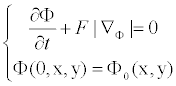(1)

Where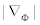denotes the normal direction,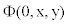is the initial contour and F represents the comprehensive forces . The advancing force F has to be regularized by an edge indication function g in order to stop the level set evolution near the optimal solution: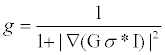(2)

Where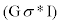is the convolution of the image I with a smoothing Gaussian kernel Gσ. A popular formulation for level set segmentation is :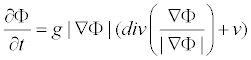(3)

Where div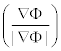approximates mean curvature k and v is a customable balloon force.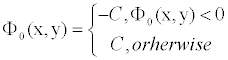(4)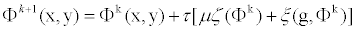(5)

In general, the level set segmentation algorithm start by using an arbitrary boundary initialization corresponding to a binary region. The thresholding algorithm proposed in this paper, automates the initialization and the parameter configuration of the level set segmentation phase . It is therefore convenient to initiate the level set function as: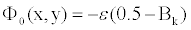(6)

Where ε is a constant regulating the Dirac function and Bk is a binary image obtained . The Dirac function is then defined as follows: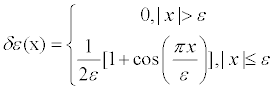(7)

Given the initial level set function ϕ0 from thresholding as in Equation. (6), it is convenient to estimate the length l and the area α by: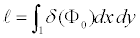(8)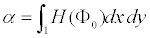(9)

Where the Heaviside function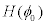is: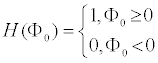(10)

The advantages of using this approach for skin lesion segmentation is that it has capabilities to separate heterogeneous objects, insensitive to noise, and automatic convergence along with control of overlapping contours into some extent.

A lesion is segmented with the initial detection of a lesion shape within a skin image by performing a thresholding method on the luminance values of the original color image. It is performed by calculating the mini–max intensity histograms on this luminance image. Finally, by using these mini–max intensity values, it computes threshold values used for detection of a lesion shape. Figure 2 illustrates this process. First we convert the RGB color image Figure 2(a) into the greyscale image Figure 2(b). By calculating the histogram thresholding, we obtain the segmented binary image as shown in Figure 2(c). In this image, the tumors represent 0 intensity values, while a background is represented by 1 value. Therefore, we can initialize a level set curve closer towards the tumors as displayed in Figure 2(d).

Figure 2: Level set initialization through adaptive thresholding: (a) original input RGB image after preprocessing steps, (b) convert into greyscale image, (c) initial tumors boundaries determine by thresholding, and (d) initialize contour.

Extraction of attributes

This step aims to design a set of robust parameters that accurately describe each lesion and this in order to ensure that melanoma and benign lesions can be distinguished (Figure 3) We used the ABCD rule in order to help the doctors to distinguish between these different tumors .

• A: Asymmetry

• B: Border irregularity

• C: Color

• D: Diameter

Asymmetry index

Asymmetry (A) is one of the more important parameters used in differentiating malignant tumors from benign lesions. A method of asymmetry index computing based on the principal axes of the lesion was proposed by Stoecker et al. . In this paper, to calculate this parameter, we determined the center symmetry through a 180° angle of rotation from first axe and second axe. Let A(x,y) be the initial surface, and B(x, y) the surface we obtained after symmetry. The ratio between the intersection of A(x, y) surfaces and B(x, y) surfaces and their merging quantifies the recovery rate of the two surfaces, and therefore the degree of symmetry (equation 17). The calculation of this index is illustrated in Figure 4, where the region in blue refers to the intersection of the surfaces and the external line defines their merging. The more the index approaches 1, the more the lesion will be considered as symmetrical.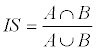(11)

Border irregularity

We can also use the border irregularity in order to give an overview of the edge type that can be found. The irregularity parameter in a lesion was presented as a very important factor when evaluating a malignant lesion. In this section, we used four special features to quantify irregular edges: compactness, radial variance and extraction of small changes in the contour.

Index compact: The compactness is implemented according to equation (12). It is evaluated on circles of different sizes, the compactness (c) is equal to 1.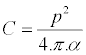(12)

Where p and a represent the perimeter and area of the lesion respectively. The lesion borders L are also evaluated using the fractal dimension D [28,29]. The lesion (L) is represented by a binary mask, consequently the object is designated by1, and the bottom by 0.

Radial variance: A lesion with irregular border has a large variance in the radial distance (the distance between its centroid G and a boundary point C). The border irregularity is estimated by the variance of the radial distance distribution.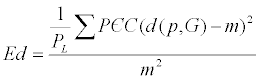(13)

Where m present the average distance d between the boundary points and the centroind G. From the distance Ed, we draw a circle that represents the radial distribution of the tumor. To quantify the border parameter, we calculate the ratio between the area of the new circle and the surface of the tumor (Figure 5).

Figure 5: Draw of the new circle, a)New circle, b)Superposition of the two edges (new circle and the initial contour).

Color criterion

Our objective in this section is to detect the color information contained in the lesion. The methodology that we propose embraces texture and form parameters in order to achieve the better classification results. Texture information is an important and efficient measure used to estimate the structure, orientation, roughness, or regularity of various regions in a set of images that enables us to distinguish between different objects . In our work we selected four parameters (Correlation, Homogeneity, Energy and Contrast) from the 14 proposed by Haralick et al. : Parameters Extracted from Haralick’s Textural Features are:

Correlation: this attribute evaluate the color distribution on the lesion. It consists in describing the evaluation of the color level from the centroid towards the boundary of a lesion.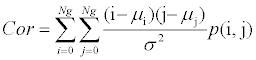(14)

Colors homogeneity: this parameter indicates a measurement of the uniformity of the grey levels of the image.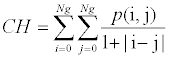(15)

Energy: This parameter has a low value when the p(i, j) have very close values and a great value when done values are large and the other small ones.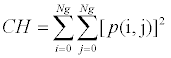(16)

Contrast: This parameter has a important numerical value if the p(i, j) are concentrated except the diagonal.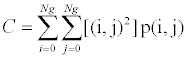(17)

Diameter: The diameter (long axis of the lesion) is one of the ABCD criteria. The algorithm that we propose for calculating the diameter is presented as follows:

➢ Image segmentation (edge extraction).

➢ Determine the coordinates(x, y) of each pixel of the lesion perimeter.

➢ Determine the coordinates(x, y) of each pixel of the lesion perimeter.

➢ The maximum of these distances is the diameter (Figure 6).

Lesions classification

We have seen that, in addition to the difficulty of standardizing the diagnostic criteria and the wide variability of the encountered structures, discrimination of certain types of lesion remains problematic. A classification system that allows tumors discrimination and analysis would be useful, especially for general practitioners who do not often observe melanomas. Such a system is introduced in Figure 7, which presents a general methodology based on the extraction of pertinent parameters. In the previous steps we have calculated a set of values that allows describing the tumor by a number of characteristics established by dermatologists. In order to classify the tumor as melanoma or benign, a multilayer neural network with supervised learning algorithms is used .

In our case, the classifier architecture is defined by different entry units representing different attributes describing the tumors (Asymmetry index, Compactness, Radial variance, Colors (Correlation, homogeneity, Energy, Contrast) and Diameter) [33,34].

In this paper, we used ANFIS classifiers for the recognition of malignant melanoma. ANFIS is an adaptive network which permits the usage of neural network topology together with fuzzy logic. It not only includes the characteristics of both methods, but also eliminates some disadvantages of their lonely-used case. Actually, ANFIS is like a fuzzy inference system with this difference that here by using feed-forward back propagation tries to minimize error. Consequent parameters are calculated forward while premise parameters are calculated backward.

The ANFIS first introduced by Jang in 1993 . It is a model that maps inputs through input membership functions (MFs) and associated parameters, and then through output MFs to outputs. The initial membership functions and rules for the fuzzy inference system can be designed by employing human expertise about the target system to be modeled. ANFIS can then purify the fuzzy if–then rules and membership functions to describe the input–output behavior of a complex system. Jang showed that even if human expertise is not available it is possible to intuitively set up practical membership functions and employs the neural training process to generate a set of fuzzy if–then rules that approximate a desired data set [33-37].

Five layers are used to create this inference system. Each layer involves several nodes described by node function.

The output signals from nodes in the previous layers will be accepted as the input signals in the present layer. After manipulation by the node function in the present layer will be served as input signals for the next layer. Here square nodes, named adaptive nodes, are adopted to represent that the parameter sets in these nodes are adjustable. Whereas, circle nodes, named fixed nodes, are adopted to represent that the parameter sets are fixed in the system. For simplicity to explain the procedure of the ANFIS, we consider two inputs x, y and one output f in the fuzzy inference system. And one degree of Sugeno’s function  is adopted to depict the fuzzy rule. Hence, the rule base will contain two fuzzy if–then rules as follows:

Rule 1: if x is A1 and y is B1 then f = p1x + q1y + r1.

Rule 2: if x is A2 and y is B2 then f = p2x + q2y + r2.

Then the frameworks of ANFIS will be able to build as shown in Figure 8. The node function in each layer is described below.

Layer 1: Each node in this layer is an adjustable node, marked by square node, with node function as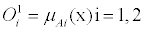(18)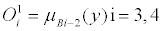(19)

Where x (or y) is the input of node, Ai (or Bi−2) is the linguistic variable. The membership function usually adopts bell-shape with maximum and minimum equal to 1 and 0, respectively.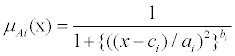(20)

Where {ai, bi, ci} represents the parameter set. It is considerable that if the values of these parameters set changes, the bell-shape function will be changed accordingly. Meanwhile, the membership functions are also different in linguistic label A. The parameters in this layer are called as premise parameters.

Layer 2: Each node in this layer is a fixed node, marked by circle node, with node function to be multiplied by input signals to serve as output signal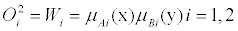(21)

The output signal Wi means the firing strength of a rule.

Layer 3: Each node in this layer is a fixed node, marked by circle node, with node function to normalize firing strength by calculating the ratio of this node firing strength to the sum of the firing strength: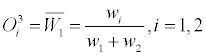(22)

Layer 4: Each node in this layer is an adjustable node, marked by square node, with node function as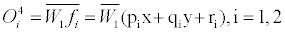(23)

Where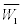is the output of layer 3, {pi, qi, ri} is parameter set which is referred as the consequent parameters.

Layer 5: Each node in this layer is a fixed node, marked by circle node, with node function to compute the overall output by: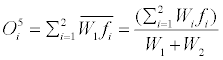(24)

Explicitly, this layer sums the node’s output in the previous layer to be the output of the whole network. From the frameworks of ANFIS, it is observed that if the parameters in the premise part are fixed, the output of the whole network system will be the linear combination of the consequent parameters, i.e.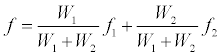(25)

Based on this characteristic, the node outputs go forward till layer 4, the resulting parameters can be identified by the least square method in the forward learning. On the other hand, the error signal go backward till layer 1, the premise parameters can be updated by the descent gradient method in the backward learning. This learning procedure is referred as hybrid-learning. The merit of hybridlearning procedure is that it can efficiently obtain the optimal premise parameters and consequent parameters in the learning process [33,34] (Figure 8).

Several fuzzy inference systems have been described by different researchers [38,39]. The most common used systems are the Mamdani type and Takagi- Sugeno type. In our work, we use zero-order Takagi- Sugeno fuzzy inference system, where the premise part of fuzzy rule is fuzzy proposition and the conclusion part is a constant. The advantage of this type is clear, because it gives a powerful tool for data classification. Output variables are obtained by applying fuzzy rules to fuzzy sets of input variables.

#### Experimental Results

We use for our experiments, 320 color images representing melanoma and benign lesions . This clinical online database of dermoscopic and clinical view lesion images were obtained from various sources but most images came from the Department of Dermatology, Health Waikato New Zealand . The images have been stored in the RGB color format of variable dimensions. The database is subdivided into six categories: 50 benign melanocytic lesions, 70 malignant melanoma lesions, 40 basal cell carcinoma lesions, 40 merkel cell carcinoma lesions, 90 seborrhoeic keratosis lesions and 30 non-melanocytic lesions. In this paper a set of discriminant features composed of four attribute attributes (A: asymmetry, B: border, C: color and D: diameter) are extracted. These attributes contain sufficient information to differentiate a melanoma from benign lesions. Finally, these various signs of specific lesion (ABCD) are provided to the proposed ANFIS classifiers in order to differentiate between malignant tumors and benign lesions (Figure 9).

For example, we note that the malignant tumors have the highest asymmetry index generally greater than 1, and it is less than one for the benign lesions. On other hand, the malignant tumors have the highest compact greater than 1 and it is less for the benign lesions. The last parameter, the malignant tumors have containing many colors. The neuro-fuzzy model classifier generates automatically a knowledge base (32 fuzzy rules) to justify the classification:

➢ If((Asymmetry index is low) and (Index compact is low) and (radial variance is low) and (colors is low) and (diameter is low)) then (output is out1mf1)

➢ If((Asymmetry index is low) and (Index compact is low) and (radial variance is low) and (colors is low) and (diameter is high)) then (output is out1mf2)

➢ …………..

➢ If((Asymmetry index is high)) and (Index compact is high)) and (radial variance is high)) and (colors is high)) and (diameter is high)) then (output is out1mf32)

The performances of the model classifier were evaluated by computing the percentages of correct rate (CC), sensitivity (SE) and specificity (SP), the respective definitions are as follows:

CC=100 *(TP+TN)/(TP +FN+TN+FP)

Se = 100 *TP/(TP +FN) is the fraction of real events that are correctly detected among all real events.

Sp = 100 *TN/(TN + FP) is the fraction of nonevents that has been correctly rejected. In these formulas TP was the number of true positives, TN was the number of true negatives, FN was the number of false negatives, and FP was the number of false positives.

Finally, we compare these results of classification obtained by ANFIS with SVM (support vector machine) and artificial neural network. The network architecture (ANN) input’ is defined by these entry units. The obtained results are summarized in Table 1 for two numbers of hidden units so that the effect of architecture on the performance could be assessed. This table records correct detection rates, using the training set. Accuracy of classification on the testing set is evaluated in terms of sensitivity Sn (percentage of malignant lesions correctly classified) and specificity Sp (percentage of benign lesions correctly classified). For each hidden units, the network weights are initialized randomly over (0,1) in every execution. The final results, given in Table 1, are calculated as the average over a set of 100 executions. In conclusion, given the disposed database, the perceptron with two hidden layers leads to better results with correct classification rate of 87.32%, sensitivity (Sn) of 90.34% and specificity (Sp) of 33.29%.

classifier Correct rate(%) Se(%) Sp(%)
SVM 98.33 96.67 90.23
ANFIS 92.31 89.13 100
ANN 87.32 90.34 33.29

Table 1: Performances of the neuro-fuzzy model classifier with ANN and SVM.

We see clearly from Table 1, that the tree approaches provide a good accuracy. Generally, we obtain the high accuracy with the ANN classifier. On the other hand, the results obtained by neuro-fuzzy model classifier are explicit and interpretable.

#### Conclusion

In the present paper we proposed several algorithms for the segmentation and characterization of dermatological images. Our objective was to determine the information referenced by dermatologists, and we were able to demonstrate the feasibility of this approach by creating prototypes capable of recognizing an indicator. In this work, we studied melanoma of the skin by means of image processing techniques and classification methods. We started with a preprocessing step based on a median filter and the DullRazor technique for its ability to remove the noise. In the second step, a segmentation approach was proposed in order to accurately locate and isolate the lesions. In this paper, an automatic method based on thresholding and level set for segmentation of skin cancer images was presented. Then, a new system for characterizing digital images of skin lesions has been presented in the paper. A sequence of transformation was applied to the lesion in order to extract its different attributes (ABCD). Series of experiments have been performed to calculate the different asymmetric measurements for the digitized color images of lesions. The results of the present study are significant and quite promising for the future. The final operation was aiming to construct a classifier used with several criteria allowing the diagnosis to be evaluated. In this paper, we compared the results of classification obtained by ANFIS with SVM and artificial neural network, and discuss how these results may influence in the following steps: the feature extraction and the final lesion classification.

#### References

Select your language of interest to view the total content in your interested language

### Article Usage

• Total views: 12474
• [From(publication date):
October-2014 - Nov 22, 2019]
• Breakdown by view type
• HTML page views : 8649Can't read the image? click here to refresh